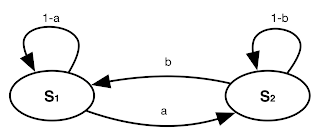## Sunday 17 January 2016

### Introduction to Transition matrix and Superposition of states

Consider the following simple state transition network, it is just a simpler version of what we had in our previous post. In each state, we have only two options either staying in the state or going out to the other state. So it is obvious that if we consider the probability of going from S1 to S2 as a, the probability of staying in S1 would be 1-a. Same for when you are in S2, you either go to S1 with the probability of b or stay there with the probability of 1-b.Simple 2 states transition network

At any given time like t, you are either in S1 or S2 so if we consider S(t) as the current state which is a vector of probabilities of being in S1 or S2  while we are in time t like bellow.State of the system at time t

This is something like the quantum superposition, you may like to read about the Schrödinger's cat, when a cat can be both alive or dead! or read about Quantum Superposition. Anyway, at any given time your system could be either in S1 or S2  each with a probability. Now the question is based on the state transition network we have, what will be the system's state at t+1 orS(t+1)? Consider the following matrix which we call it transition matrix:Transition Matrix based on the drawn network

OK, it is simple, considering this transition matrix based on the network we have, the next state of the system can be derived from this simple formula S(t+1) = T x S(t) or:Calculating the state of the system at t+1

Note that here time is a discrete quantity so t+1 just means next quantum of time after t. Now let us see what this multiplication means. Suppose you are 100% sure that at time t the system is absolutely in state S1, in this case P(S1) = 1 and P(S2) = 0 and the new state vector will be something like the following which completely makes sense based on the graph:An special case in which we are 100% sure the system is in S1 so P(S1) = 1 and P(S2) = 0

You can also easily see how it is when P(S1) = 0 and P(S2) = 1 and the middle points too, where you really don't know the exact state of the system. So you see we can easily find the state of the system at time t+1 and even more! Based on the new state (or you may say new superposition state) we can find the state of the system at t+2 like S(t+2) = T x S(t+1) and S(t+3) = T x S(t+2) and ...

So it seems that we can predict the future, can't we? Yes and No. Yes because as you see for this simple system the calculation is so easy and you can find S(t+n) for any given n just based on your current state. And no, because first you can't directly jump and make some calculation to derive S(t+n) you need to do n times matrix multiplication and it is also gets more complicate when you are dealing with practical systems with some high dimensional state vectors and transition matrix. But in theory it is true, you can go forward as much as you like and predict the future states.

And finally for this simple example since P(S1)+P(S2)=1 and 1-a=b and 1-b=a then the calculation result can be simplified, as a matter of fact if you do this you'll find that P(S1) will be b  and P(S2) will be a at any time after t0, regarding of the initial values of P0(S1) and P0(S2)!